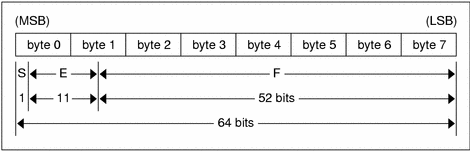ONC+ Developer's Guide

## Floating Point

The standard defines the floating-point data type `float` (32 bits or 4 bytes). The encoding used is the IEEE standard for normalized single-precision floating-point numbers . The following three fields describe the single-precision floating-point number:

S: The sign of the number. Values 0 and 1 represent positive and negative respectively. One bit.

E: The exponent of the number, base 2. Eight bits are in this field. The exponent is biased by 127.

F: The fractional part of the number's mantissa, base 2. Twenty-three bits are in this field.

Therefore, the floating-point number is described by.

`(-1)**S * 2**(E-Bias) * 1.F `

### Declaration

Single-precision floating-point data is declared as follows.

`float identifier; `

Double-precision floating-point data is declared as follows.

`double identifier; `

### Double-Precision Floating Point EncodingJust as the most and least significant bytes of an integer are 0 and 3, the most-significant and least-significant bits of a double-precision floating-point number are 0 and 63. The beginning bit, and most significant bit, offsets of S, E, and F are 0, 1, and 12 respectively.

These offsets refer to the logical positions of the bits, not to their physical locations, which vary from medium to medium.

Consult the IEEE specifications about the encoding for signed zero, signed infinity (overflow), and de-normalized numbers (underflow) . According to IEEE specifications, the NaN (not a number) is system dependent and should not be used externally.